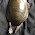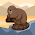## Wednesday, April 8, 2015

### 什么是强化学习？ (What is reinforcement learning?)

Reinforcement learning 是机器学习里面的一个分支，特别善於控制一只能够在某个环境下 自主行动 的个体 (autonomous agent)，透过和 环境 之间的互动，例如 sensory perception 和 rewards，而不断改进它的 行为

## 输入／输出

• 状态 (States) = 环境，例如迷宫的每一格是一个 state
• 动作 (Actions) = 在每个状态下，有什么行动是容许的
• 奖励 (Rewards) = 进入每个状态时，能带来正面或负面的 价值 (utility)

• 方案 (Policy) = 在每个状态下，你会选择哪个行动？

1. states S = 迷宫中每一格的位置，可以用一对座标表示，例如(1,3)
2. actions A = 在迷宫中每一格，你可以行走的方向，例如：｛上，下，左，右｝
3. rewards R = 当前的状态 (current state) 之下，迷宫中的那格可能有食物 (+1) 、也可能有怪兽 (-100)
4. policy P = 一个由 状态 $\rightarrow$ 行动 的 函数，意即： 这函数对给定的每一个状态，都会给出一个行动。
（S, A, R）是使用者设定的， P 是算法自动计算出来的。

## 程式

https://studywolf.wordpress.com/2012/11/25/reinforcement-learning-q-learning-and-exploration/

def learnQ(self, state, action, reward, value):
oldv = self.q.get((state, action), None)
if oldv is None:
self.q[(state, action)] = reward
else:
self.q[(state, action)] = oldv + self.alpha * (value - oldv)

## 強化學習的原理

《AI－a modern approach》这本书第 21 章有很好的简介。  《AIMA》自然是经典，很多人说他们是读这本书而爱上 AI 的。  这本书好处是，用文字很耐性地解释所有概念和原理，思路很清晰，使读者不致有杂乱无章的感觉。  例如 21 章 首先讲 passive reinforcement learning，意思是当 policy 是固定时，纯粹计算一下 agent 期望的价值（utility，即 rewards 的总和） 会是多少。  有了这基础后再比较不同 policies 的好坏。  这种思路在数学中很常见： 首先考虑简单到连白痴也可以解决的 case，然后逐步引入更多的复杂性。  例如数学归纳法，由 N=1 的 case 推到 N→∞ 。

## Utility (价值，或效)

U 是一连串行动的 rewards 的总和。  例如说，行一步棋的效用，不单是那步棋当前的利益，还包括走那步棋之后带来的后果。  例如，当下贪吃一只卒，但 10 步后可能被将死。  又或者，眼前有美味的食物，但有些人选择不吃，因为怕吃了会变肥。

$$U(S_0) = \mathbb{E}[ \; \sum_{t=0}^{\infty} \; \gamma^t \; R(S_t) \; ]$$

## Bellman condition

Bellman equation 说的是： 「如果从最佳选择的路径的末端截除一小部分，馀下的路径仍然是最佳路径。

（例如，你从香港乘车到北京，选择了最便宜的路线，此路线经过 10 个车站，第二站是深圳：
$$\mbox{香港} \rightarrow \mbox{深圳} \rightarrow ... ... \rightarrow \mbox{北京}$$

$$\mbox{深圳} \rightarrow ... ... \rightarrow \mbox{北京}$$

$$U^*(S) = \max_a \{ R(a) + U^*(S') \}$$
$$U^*(\mbox{全路径}) = \max_a \{ R(\mbox{在当前状态下选取 a}) + U^*(馀下路径) \}$$
$*$ 表示「最优 (optimal)」。 这条看似简单的式子是动态规划的全部内容； 它的意义是： 我们想获得最佳效益的路径，所以将路径切短一些，於是问题化解成一个较小的问题；  换句话说它是一个 recursive relation

## Delta rule

$$\mbox{当前状态} := \mbox{当前状态} + \alpha ( \mbox{理想} - \mbox{当前状态})$$

（Delta rule 的微分形式就是我们熟悉的「梯度下降」： $x \mbox{ += } \eta \cdot \frac{dy}{dx}$）

## Temporal difference (TD) learning

$$U(S) = R(S) + \gamma \sum_{S'} P(S \rightarrow S') \; U(S')$$

$$U(1,3) = -0.04 + U(2,3)$$

TD learning 的思想是：  假设其他 U(S') 的值正确，利用 Bellman optimality 来调整当下 state 的 U(S)。 当尝试的次数多了，所有 U 值都会趋向理想。 Agent 只需要用这条 update rule：
$$U(S) \mbox{ += } \alpha ( \; R(S) + \gamma U(S') - U(S) \; )$$

$\alpha$ 是 learning rate，它决定学习的速度（但它不能太大，避免 overshooting）。  后面那东西是 U(S) 和 U(S) 的估值 (estimation) 之间的差别。  对於理想的 U(S) 和 U(S')，那差别会是 0。  而在每个 time step，我们只是用 $\alpha$ 部分地 调整这个差别。

P 是 state transitions 的机率，换句话是关於世界的一个 model。 TD learning 不需要学习 P，所以叫 model-free learning。  但正如开篇时说过，model-free 并不一定是好事，人的智慧就是基於我们对外在世界有一些很好的 models。

## Q value

Q 值只是 U 值的一个变种 ;   U 是对每个 state 而言的，Q 把 U 值分拆成每个 state 中的每个 action 的份量。  换句话说，Q 就是在 state S 做 action A 的 utility。

Q 和 U 之间的关系是：
$$U(S) = \max_A Q(A, S)$$

Q 的好处是什么？  下面将会介绍 active learning，而 Q value  配合 TD learning，可以在 active learning 中也消除 P，达到 model-free 的效果。

$$U(S) \mbox{ += } \alpha ( \; R(S) + \gamma \max_{A'} Q(A', S') - Q(A, S) \; )$$

## Active learning

Agent 需要尝试一些未知的状态／行动，才会学到 optimal policy；  这就是所谓的 exploration vs exploitation （好奇心 vs 短暂贪婪）之间的平衡。

$$U(S) = R(S) + \gamma \max_A \mathcal{F}[ \; \sum_{S'} P(S \rightarrow S') U(S'), \; N(A, S) \; ]$$

## 结语

1.2.3.